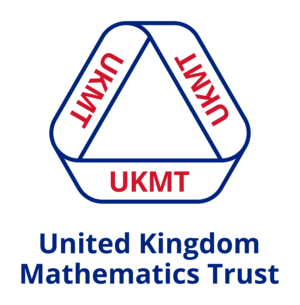## United Kingdom Mathematics Trust

The questions have been grouped into two sets of quizzes:

### 1. Themed Quizzes

These consist of sets of four or five questions grouped by topic. You may choose to use these at the end of teaching a topic unit to provide some challenge, as starters or plenaries to promote positive discussion, as group work tasks, or as extension material for some students in your class whilst you work on the fundamentals with others.

### 2. Mathematical Challenge Practice Quizzes

These are sets of five questions that each cover a random selection of topics. There are three challenge levels for these Practice Quizzes:

Bronze Taken from questions 1 to 8 on the original Maths Challenge Papers
Silver Taken from questions 9 to 16 on the original Maths Challenge Papers
Gold Taken from questions 17 to 25 on the original Maths Challenge Papers

Each quiz is taken from a range of years, so you can use these alongside the paper versions of the Mathematical Challenges. You may choose to use these in the build up to a Challenge, or make use of them throughout the year as a way of helping students develop problem solving and analytical skills.

The UKMT questions and quizzes may be used in class, or set for students to answer on our system. The advantage of the latter is that they will be marked for you, and you can access detailed insights into your students’ performance and possible misconceptions.

All these questions have been adapted from Junior, Intermediate and Senior Mathematical Challenge problems. However, they have not been labelled as such, and hence can be used by students of all ages to challenge them to think mathematically!

The UK Mathematics Trust is a registered charity that aims to advance the education of young people in mathematics.

The UK Mathematics Trust is a registered charity that aims to advance the education of young people in mathematics.They run solo and team competitions, organise enrichment activities for students, publish books, and train the UK team for the International Mathematical Olympiad. Find out more about the UKMT on their website website.

## Quizzes

Angle Problems
Arithmetic in Context (1/3)
Arithmetic in Context (2/3)
Arithmetic in Context (3/3)
Arithmetic without context
Averages
Circles
Combinations and Logical Listings (1/2)
Combinations and Logical Listings (2/2)
Coordinates
Decimals
Estimation
Factors, Multiples and Primes
Fraction, Decimal and Percentage Equivalence
Fractions (1/2)
Fractions (2/2)
Money Problems
Negative Numbers
Order of Operations (BIDMAS)
Percentages
Perimeter and Area
Place Value
Powers and Roots
Ratio
Reflection and Symmetry
Sequences
Speed
Surface Area and Volume
Time Problems
Units of Measurement
Visualising 2D Shapes
Visualising 3D Shapes
Quiz 1
Quiz 2
Quiz 3
Quiz 4
Quiz 5
Quiz 6
Quiz 7
Quiz 8
Quiz 9
Quiz 10
Quiz 11
Quiz 12
Quiz 13
Quiz 14
Quiz 15
Quiz 16
Quiz 17
Quiz 18
Angles
Arithmetic - context
Arithmetic - no context
Averages and Range
Circles
Coordinate Geometry
Decimals
Estimation
Factors, Multiples and Primes
Fractions
Geometrical reasoning
Logical reasoning
Money problems
Percentages
Perimeter and Area
Powers, Indices and Roots
Pythagoras and Trigonometry
Ratio and Proportion
Sequences
Speed, Distance, Time
Surface Area and Volume
Systematic Listing
Time Problems
Quiz 1
Quiz 2
Quiz 3
Quiz 4
Quiz 5
Quiz 6
Quiz 7
Quiz 8
Quiz 9
Quiz 10
Quiz 11
Quiz 12
Quiz 13
Quiz 14
Quiz 15
Quiz 16
Quiz 17
Angles
Arithmetic
Circles
Combinations
Coordinate Geometry
Dates and Time
Equations and Algebra
Factors, Multiples, Primes
Fractions
Percentages
Perimeter and Area
Powers and Roots
Properties of Shapes
Pythagoras and Trigonometry
Speed, Distance, Time
Surface Area and Volume
Units of measure
Quiz 1
Quiz 2
Quiz 3
Quiz 4
Quiz 5
Quiz 6
Quiz 7
Quiz 8
Quiz 9
Quiz 10